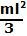## Wednesday, November 12, 2008

### Irodov Problem 1.245The force is initially applied perpendicular to the rod. This causes the rod to rotate. As the rod rotates, since the direction of F is no longer perpendicular to the rod and so the moment arm decreases. As seen in the diagram, if the rod has rotated an angle, the effective torque on the rod is only- due to the component of the force that is perpendicular to the rod. The moment of inertia of the rod about its axis of rotation (passing through one if its ends perpendicular to the plane) is. So we have,